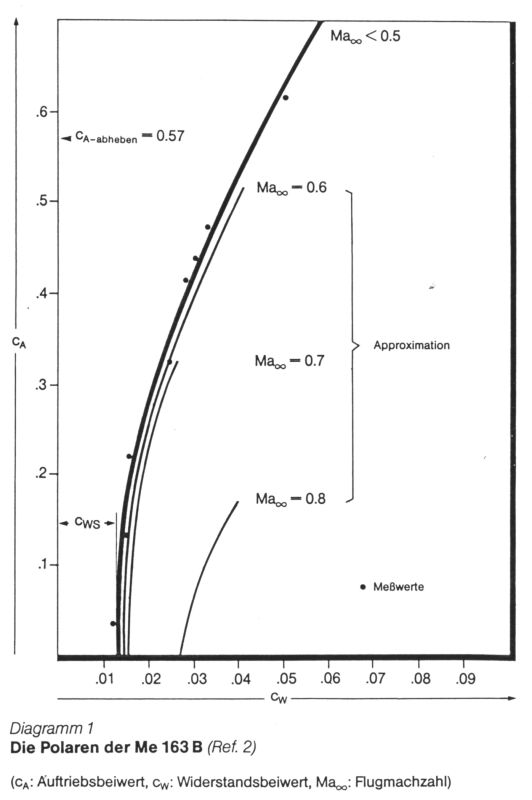# Me 163B polars

Shown here is the polar as presented in 'Der Streng Geheime Vogel Me 163' by Wolfgang Späte, Appendix C, page 309-310. It is a combination of actual measurements (source yet unknown) and calculations. The calculations for Mach 0.5 to 0.8 were made according to a method presented in 'Aircraft performance, stability and control' by Perkins and Hage, Wiley, New York, 1963. The graph identifies the polars as approximations. The graph identifies CL=0.57 as the lift-off value.

According to the accompanying text, the basic polar (Mach below 0.5) is given by the standard equation CD = CD0 + CL^2 / (pi*A*e). CD0 is 0.013, aspect ratio 4.41, Oswald correction factor 0.786. The aspect ratio figure is based on wing surface figure of 19.6 m^2, while I keep measuring 17.4 m^2, using the standard definition of wing area of extending the leading and trailing edges to the centerline. Possibly the gross area (wing plus fuselage) is 19.6 m^2. Using a wing area figure of 17.4 m^2, I calculate an aspect ratio of 5.0.

Measurement of the data points and graphs below.The data points were measured digitally.

 Drag coefficient Lift coefficient 0.0122 0.033 0.0151 0.131 0.0158 0.218 0.0248 0.321 0.0284 0.411 0.0305 0.435 0.0333 0.469 0.0505 0.611

The curves were measured and the equations below were the calculated as best fit equations.

 M<0.5 CD = 0.0130 + 0.0953 * CL^2 M=0.6 CD = 0.0141 + 0.1037 * CL^2 M=0.7 CD = 0.0154 + 0.1015 * CL^2 M=0.8 CD = 0.0283 + 0.4473 * CL^2